# SAT Math Multiple Choice Question 177: Answer and Explanation

### Test Information

Question: 177

12. The cost in dollars, C, of producing a custom-made T-shirt with a team logo is given by the formula C = 110 +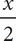, where x is the number of T-shirts produced. When every T-shirt produced is sold, the revenue from selling the customized T-shirts is given by R = 15x -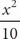. Which one of the following would be the formula for the profit from producing and selling x T-shirts?

(Profit = Revenue - Cost)

• A. --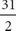x + 110
• B. --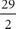x + 110
• C. -+x - 110
• D. -+x - 110

C Whenever there are variables in the question and in the answers, think Plugging In. If x = 10, then C = 110 +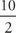= 110 + 5 = 115 and R = 15 (10) -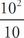= 150 -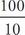= 150 - 10 = 140. Therefore, the profit can be calculated as 140 - 115 = 25. Plug 10 in for x in the answers to see which answer equals the target number of 25. Choice (A) becomes --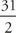(10) + 110 = -- 31 (5) + 110 = -10 - 155 + 110 = -55. This doesn't match the target number, so eliminate (A). Choice (B) becomes --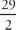(10) + 110 = -- 29(5) + 110 = -10 - 145 + 110 = -45. Eliminate (B). Choice (C) becomes -+(10) - 110 = -+ 29(5) - 110 = -10 + 145 - 110 = 25. Keep (C), but check (D) just in case it also works. Choice (D)becomes -+(10) - 110 = -+ 31(5) - 110 = -10 + 155 - 110 = 35. Eliminate (D) and choose (C).